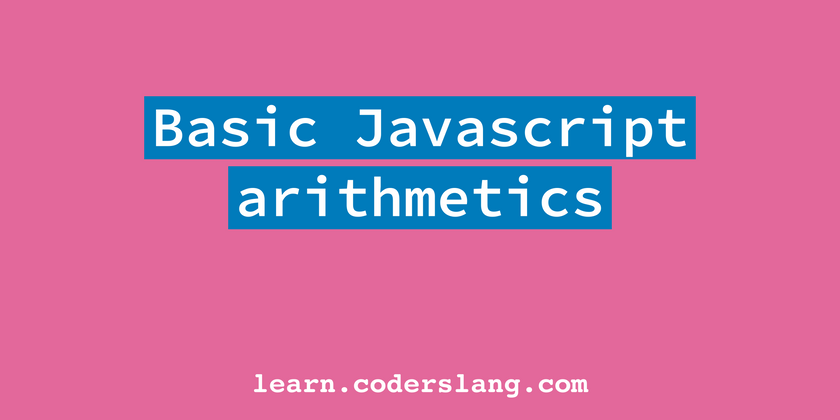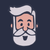## CodeNewbie Community is a community of 3,206 amazing newbies

Log in to customize your experience and get involved.

loading...# Basic math operations in JavaScriptCoderslang: Become a Software Engineer 🚀 Originally published at learn.coderslang.com ・3 min read

JavaScript supports all basic arithmetic operations. In this guide, we'll dive into them and see how do the regular `+`, `-`, `*`, and `/` perform on different data types.

## Addition

You can use the binary operator `+` to add two numbers in JS. It’s called binary because it needs exactly two operands (numbers in our case) to perform the desired action.

``````const sum = 2 + 2;                          // 4
``````

So, the result is exactly what you would expect here, but things change when we start using other data types. For example, if one of the operands is a string, then the other one will also be considered a string. Those two strings will be concatenated or "glued" together.

``````const numberPlusString = 2 + '2';            // 22
const booleanPlusString = true + 'Story';    // trueStory
const stringPlusString = 'just' + 'Strings'; // justStrings
``````

You can also place a regular object, an array, or a function on any side of the `+` operator. In this case, they will be first converted to a string and then the addition will be done.

``````const f = () => {return 0};
const obj = {type: 'regular'};
const arr = [1, 2, 3];

console.log('Hello!' + f);                   // Hello!() => {return 0}
console.log(true + obj);                     // true[object Object]
console.log(1 + arr);                        // 11,2,3
``````

Notice that most objects will be converted to strings as `[object Object]`. If you want to do something different, then you should implement a custom `toString()` function.

``````const obj = {
type: 'regular',
toString: function () {
return JSON.stringify(this);
},
};

console.log(1 + obj);                         // 1{"type":"regular"}
``````

Interesting things happen when either both operands are boolean or one of them is a boolean and another one is a number. In this case `true` will always be converted to `1` and `false` will become `0`.

``````const truePlusTrue = true + true;             // 2
const truePlusFalse = true + false;           // 1
const booleanPlusNumber = true + 5;           // 6
``````

## Subtraction, multiplication, and division

Even though the rules for addition might seem quite complex, other basic operations follow common sense logic. With numbers, everything is as expected.

``````const subtractionResult = 10 - 2;              // 8
const multiplicationResult = 2 * 2;            // 4
const divisionResult = 10 / 2;                 // 5
``````

Booleans are still converted to either `0` or `1` when on the other side is a boolean or a number.

``````console.log(true / true);                      // 1
console.log(5 * false);                        // 0
console.log(true - false);                     // 1
``````

### `Infinity` and `-Infinity`

If you try to divide something by `0` or `false`, then the result is either `Infinity` or `-Infinity`.

``````console.log(5 / 0);                             // Infinity
console.log(-5 / false);                        // -Infinity
``````

### NaN

In most other cases when it’s hard to make sense of the arithmetic expression, the result will be `NaN` or "not-a-number".

``````console.log(false / false);                     // NaN
console.log(10 / 'string');                     // NaN
console.log(5 * {});                            // NaN
console.log({} - [])                            // NaN
``````

### Empty Array

An empty array is converted either to an empty string or into `0` whenever possible.

``````console.log('str1' + [] + 'str2');               // str1str2
console.log(12 * []);                            // 0
console.log(5 - []);                             // 5
console.log(1 / []);                             // Infinity
``````

## Unary increment and decrement

Two very useful operators allow you to either increment or decrement the value of the variable by 1. They look like double plus `++` and double minus `--`.

``````let counter = 0;
counter++;
console.log(counter);                             // 1
counter--;
console.log(counter);                             // 0
``````

The `++` and `--` operators can be placed on either side of the variable. Both `counter++` and `++counter` expressions are valid. The difference can be represented by these examples:

``````let i = 0;
console.log(i++);                                 // 0
console.log(i);                                   // 1
``````

So, first, we’ve taken the value of `i`, logged it into the screen, and then did the increment, which we see in the second `console.log`.

With `++i` it’s the other way around.

``````let i = 0;
console.log(++i);                                  // 1
console.log(i);                                    // 1
``````

To make sure you got this right, please answer the following question in the comments below.

``````let x = 1;
let y = 2;
let z = 3;

console.log(++x - y-- + z++);                       // ?
``````

## Conclusion

We’ve covered the basic arithmetic operations in JavaScript, their rules, and exceptions.

The `+`, `-`, `*`, `/` behave as expected with numbers, but with strings, objects, arrays, functions, and booleans it changes a lot.

Learning the fundamentals of JS (like math) is useful for any web developer.

Read more JavaScript tutorials or Learn Full-Stack JS from scratch!